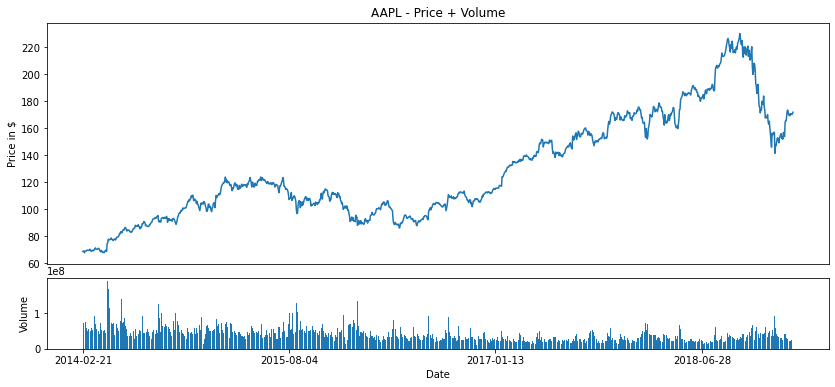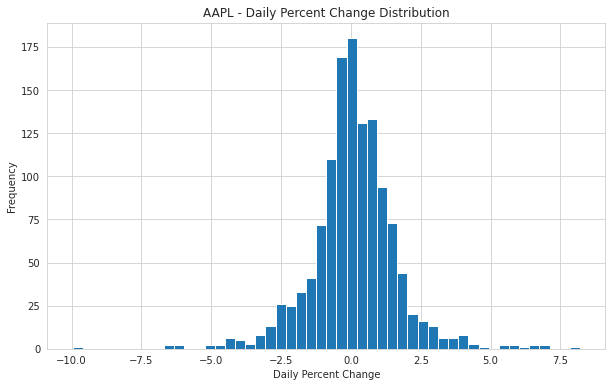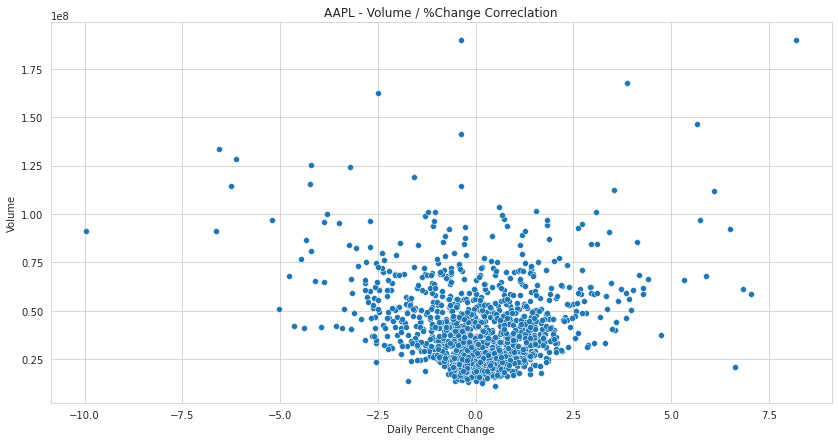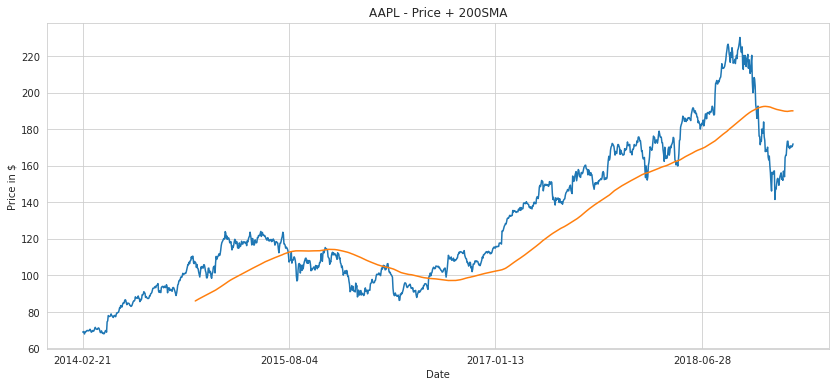## Apple stock price analysis

In this project we will analyse Apple'stock price from 2014 to 2019 and try to see if there are any trading strategy that we could use for long term investors and for traders.

To start, we will explore AAPL data downloaded from Kaggle. We will preview the data first with very basic built-in functions, then we will try to go deeper and answer some questions in relation to Apple' stock price using visualization and other tools. We will finally draw our conclusion.

#### How to run the code

This is an executable Jupyter notebook hosted on Jovian.ml, a platform for sharing data science projects. You can run and experiment with the code in a couple of ways: using free online resources (recommended) or on your own computer.

##### Option 1: Running using free online resources (1-click, recommended)

The easiest way to start executing this notebook is to click the "Run" button at the top of this page, and select "Run on Binder". This will run the notebook on mybinder.org, a free online service for running Jupyter notebooks. You can also select "Run on Colab" or "Run on Kaggle".

##### Option 2: Running on your computer locally
1. Install Conda by following these instructions. Add Conda binaries to your system `PATH`, so you can use the `conda` command on your terminal.

2. Create a Conda environment and install the required libraries by running these commands on the terminal:

``````conda create -n zerotopandas -y python=3.8
conda activate zerotopandas
pip install jovian jupyter numpy pandas matplotlib seaborn opendatasets --upgrade
``````
1. Press the "Clone" button above to copy the command for downloading the notebook, and run it on the terminal. This will create a new directory and download the notebook. The command will look something like this:
``````jovian clone notebook-owner/notebook-id
``````
1. Enter the newly created directory using `cd directory-name` and start the Jupyter notebook.
``````jupyter notebook
``````

You can now access Jupyter's web interface by clicking the link that shows up on the terminal or by visiting http://localhost:8888 on your browser. Click on the notebook file (it has a `.ipynb` extension) to open it.

Let's install the necessary packagess

In :
``````!pip install jovian opendatasets --upgrade --quiet
``````

Now let's download the data, and list the files within the dataset.

In :
``````# Dataset URL
dataset_url = 'https://www.kaggle.com/timoboz/stock-data-dow-jones' ``````
In :
``````# kaggle key : fc36bfb9b99ec255ca263243a5b5ebbb
import opendatasets as od
``````
```Please provide your Kaggle credentials to download this dataset. Learn more: http://bit.ly/kaggle-creds Your Kaggle username: quentdcr Your Kaggle Key: ········ ```
`100%|██████████| 1.62M/1.62M [00:00<00:00, 39.3MB/s]`
```Downloading stock-data-dow-jones.zip to ./stock-data-dow-jones ```
``` ```

In :
``````# File Directory
data_dir = './stock-data-dow-jones'``````
In :
``````import os
os.listdir(data_dir)
``````
Out:
``````['AAPL.csv',
'JPM.csv',
'IBM.csv',
'HD.csv',
'WMT.csv',
'CVX.csv',
'BA.csv',
'TRV.csv',
'GS.csv',
'MCD.csv',
'DWDP.csv',
'INTC.csv',
'VZ.csv',
'CAT.csv',
'UNH.csv',
'MRK.csv',
'DIS.csv',
'KO.csv',
'CSCO.csv',
'MSFT.csv',
'WBA.csv',
'PG.csv',
'NKE.csv',
'PFE.csv',
'JNJ.csv',
'AXP.csv',
'V.csv',
'UTX.csv',
'XOM.csv',
'MMM.csv']``````
In :
``project_name = "AAPL-price-analysis" # change this (use lowercase letters and hyphens only)``

### Data Preparation and Cleaning

Exploring the data and checking whether the format is usable.

In :
``import pandas as pd``
In :
``````# Reading Dataframe
aapl_df = pd.read_csv(data_dir + "/AAPL.csv", parse_dates=True)
aapl_df
``````
Out:
In :
``````# Shape of Dataframe
aapl_df.shape
``````
Out:
``(1258, 12)``
In :
``````# Basic info of Dataframe
aapl_df.info()
``````
```<class 'pandas.core.frame.DataFrame'> RangeIndex: 1258 entries, 0 to 1257 Data columns (total 12 columns): # Column Non-Null Count Dtype --- ------ -------------- ----- 0 date 1258 non-null object 1 open 1258 non-null float64 2 high 1258 non-null float64 3 low 1258 non-null float64 4 close 1258 non-null float64 5 volume 1258 non-null int64 6 unadjustedVolume 1258 non-null int64 7 change 1258 non-null float64 8 changePercent 1258 non-null float64 9 vwap 1258 non-null float64 10 label 1258 non-null object 11 changeOverTime 1258 non-null float64 dtypes: float64(8), int64(2), object(2) memory usage: 118.1+ KB ```
In :
``````# Description of Dataframe
aapl_df.describe()
``````
Out:
In :
``````# Check for columns
aapl_df.columns
``````
Out:
``````Index(['date', 'open', 'high', 'low', 'close', 'volume', 'unadjustedVolume',
'change', 'changePercent', 'vwap', 'label', 'changeOverTime'],
dtype='object')``````
In :
``````# Check for NAN values
aapl_df.first_valid_index()
``````
Out:
``0``

### Exploratory Analysis and Visualization

Data analysis and visualisation of stock price, volume, and understanding of correlation.

Let's begin by importing `matplotlib.pyplot`, `numpy` and `seaborn`.

In :
``````import seaborn as sns
import numpy as np
import matplotlib
import matplotlib.pyplot as plt
import matplotlib.dates as mdate

%matplotlib inline
``````
##### Q1: What is AAPL stock general trend and volume look like ?

We will plot the closed price with the volume here.

In :
``````plt.figure(figsize=(14,6))

top = plt.subplot2grid((4,4), (0, 0), rowspan=3, colspan=4)
bottom = plt.subplot2grid((4,4), (3,0), rowspan=1, colspan=4)

top.plot(aapl_df['date'], aapl_df['close'])
bottom.bar(aapl_df['date'], aapl_df['volume'])

top.axes.get_xaxis().set_visible(False)
top.set_title('AAPL - Price + Volume')
top.set_ylabel('Price in \$')
bottom.set_ylabel('Volume')
bottom.set_xlabel('Date');

locator = mdate.YearLocator()
plt.gca().xaxis.set_major_locator(locator);
``````##### Q2: What has been AAPL yearly average return from 2014-02-21 to 2019-02-20 ?

For that question, we will first locate and define the elements needed, and then we will compute the average return using the coumpound interest formula.

In :
``````# Elements definition
initial_price = aapl_df.at[0, 'open'] # here \$69.9727
final_price = aapl_df.at[1257, 'close'] # here \$172.03

aapl_df['year'] = pd.DatetimeIndex(aapl_df.date).year # Adding "year" column
nb_of_years = aapl_df['year'].nunique() - 1 # here 5 years

# Yearly average return formula
roi = ((final_price/initial_price)**(1/nb_of_years)-1)

print("The yearly average return of Apple stock from February 2014 to February 2019 is {:0.2f}%.".format(roi*100))``````
```The yearly average return of Apple stock from February 2014 to February 2019 is 19.71%. ```
##### Q3: What does the Daily % Change distribution look like ?

For this question, we will use an histogram to better understand the distribution, and use some built in functions.

In :
``````sns.set_style('whitegrid')

plt.figure(figsize=(10,6))
plt.hist(aapl_df.changePercent, bins=50)
plt.title("AAPL - Daily Percent Change Distribution")
plt.xlabel('Daily Percent Change')
plt.ylabel('Frequency');``````In :
``print('The daily % change varies mostly between -2% and 2%.')``
```The daily % change varies mostly between -2% and 2%. ```
##### Q4: Is there a correlation between Volume and % Change ?

Here we will first calculate the correlation, then plot the values using a scatter chart.

In :
``````r = np.corrcoef(aapl_df.changePercent, aapl_df.volume)
print("The correlation coefficient between the Volume and the % Change is {:0.3f}.".format(r[0,1]))``````
```The correlation coefficient between the Volume and the % Change is -0.054. ```
In :
``````plt.figure(figsize=(14,7))
plt.title("AAPL - Volume / %Change Correclation")
plt.xlabel('Daily Percent Change')
plt.ylabel('Volume')

sns.scatterplot(x=aapl_df.changePercent, y=aapl_df.volume);
``````##### Q5: Could we implement a trading strategy using the 200SMA ?

We can try to plot the 200SMA and see if there is any interesting things.

In :
``````sma200 = aapl_df['close'].rolling(200).mean()
aapl_sma = pd.DataFrame({'SMA 200': sma200})

plt.figure(figsize=(14,6))
plt.plot(aapl_df.date, aapl_df.close, aapl_sma)
plt.title("AAPL - Price + 200SMA")
plt.xlabel('Date')
plt.ylabel('Price in \$')

locator = mdate.YearLocator()
plt.gca().xaxis.set_major_locator(locator);
``````In :
``print('Observation: The 200SMA reacts well to the price.')``
```Observation: The 200SMA reacts well to the price. ```

### Inferences and Conclusion

Through this analysis we have tried to understand some figures about Apple'stock price. We have discovered that the general trend has been bullish since 2014. We saw that he yearly average return of the stock price is around 19.7%, which is pretty attractive for long term investors. We have seen that there is not so much volatility during the day, the price moves from -2% to 2%, which is not very attractive for retail traders. We tried to see if there was any correlation between the %Change and the volume but there was not really. And finally we have seen that the 200SMA reacts extremely well to the price.

To conclude, we can use two trading strategies for Apple'stock price:

1. For long term investors: buy and hold

Submission Instructions (delete this cell)

(Optional) Write a blog post

In :
``import jovian``
In :
``jovian.commit()``
```[jovian] Attempting to save notebook.. [jovian] Updating notebook "quentinducrocq/aapl-price-analysis" on https://jovian.ai [jovian] Uploading notebook.. [jovian] Uploading additional files... [jovian] Committed successfully! https://jovian.ai/quentinducrocq/aapl-price-analysis ```
Out:
``'https://jovian.ai/quentinducrocq/aapl-price-analysis'``
In [ ]:
`` ``